# Floyd: MCQ in Basic Op-Amp Circuits

(Last Updated On: December 7, 2019)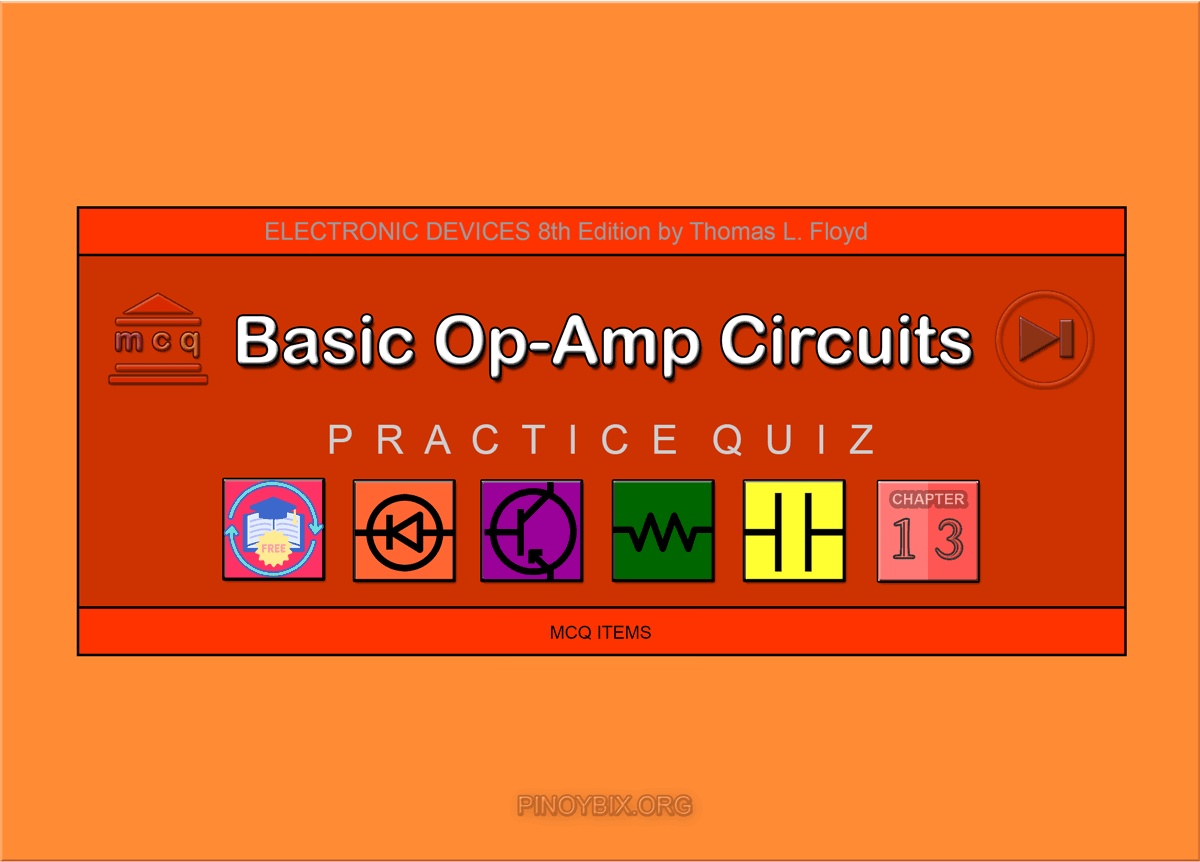This is the Multiple Choice Questions in Basic Op-Amp Circuits from the book Electronic Devices – Electron Flow Version and Conventional Current Version 8th Edition by Thomas L. Floyd. If you are looking for a reviewer in Electronics Engineering this will definitely help. I can assure you that this will be a great help in reviewing the book in preparation for your Board Exam. Make sure to familiarize each and every questions to increase the chance of passing the ECE Board Exam.

If you are looking for the Self-test in Floyd’s Electronic Devices proceed to

#### Practice Exam Test Questions

Choose the letter of the best answer in each questions.

1. If an op-amp comparator has a gain of 100,000, an input difference of 0.2 mV above reference, and a supply of ±12 V, the output will be

A. 20 V.

B. 12 V.

C. 10 V.

D. 15 V.

Solution:

2. To reduce the effects of noise resulting in erratic switching of output states of a comparator, you can use

A. the upper trigger point.

B. the lower trigger point.

C. nonzero-level detection.

D. hysteresis.

Solution:

3. A comparator with a Schmitt trigger has

A. two trigger levels.

B. a fast response.

C. a slow response.

D. one trigger level.

Solution:

4. In a comparator with output bounding, what type of diode is used in the feedback loop?

A. Schottky

B. junction

C. zener

D. varactor

Solution:

5. In a flash A/D converter, the priority encoder is used to

A. select the first input.

B. select the highest value input.

C. select the lowest value input.

D. select the last input.

Solution:

6. A differentiator is used to measure

A. the sum of the input voltages.

B. the difference between two voltages.

C. the area under a curve.

D. the rate of change of the input voltage.

Solution:

7. An op-amp has an open-loop gain of 90,000. Vsat = ±13 V. A differential voltage of 0.1 V p-p is applied between the inputs. What is the output voltage?

A. 13 V

B. –13 V

C. 13 Vp-p

D. 26 Vp-p

Solution:

8. Refer to Figure 13-1(a). Determine the output voltage.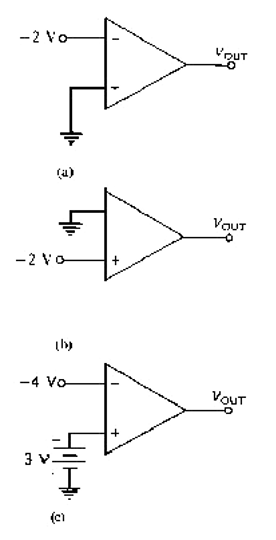Figure 13-1

A. 1 V

B. –1 V

C. +Vsat

D. –Vsat

Solution:

9. Refer to Figure 13-1(b). What is the output voltage?

A. 2 V

B. –2 V

C. +Vsat

D. –Vsat

Solution:

10. Refer to Figure 13-1(c). With the inputs shown, determine the output voltage.

A. 7 V

B. –7 V

C. +Vsat

D. –Vsat

Solution:

11. Refer to Figure 13-2(a). What is the output voltage?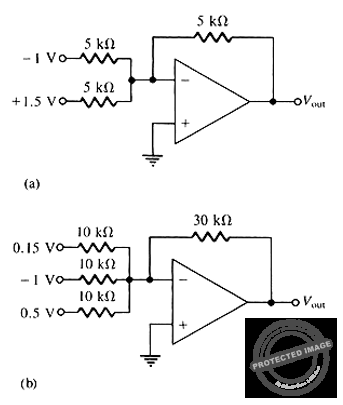Figure 13-2

A. 0.5 V

B. –0.5 V

C. 2 V

D. –2 V

Solution:

12. Refer to Figure 13-2(b). Determine the output voltage, VOUT.

A. 1.05 V

B. –0.35 V

C. 0.35 V

D. –1.05 V

Solution:

13. Refer to Figure 13-3(a). Determine the upper trigger point.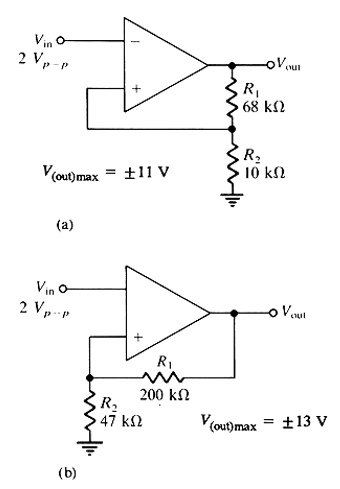Figure 13-3

A. V(out)max

B. –V(out)max

C. –1.41 V

D. +1.41 V

Solution:

14. Refer to Figure 13-3(b). Determine the lower trigger point.

A. +V(out)max

B. –V(out)max

C. –2.47 V

D. +2.47 V

Solution:

15. Refer to Figure 13-4(a). This circuit is known as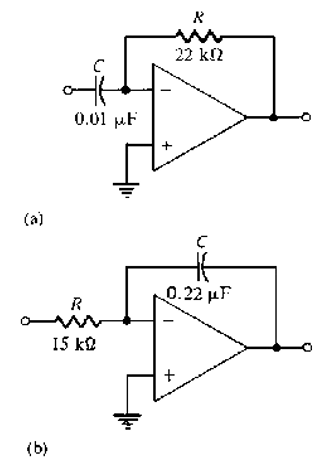Figure 13-4

A. a noninverting amplifier.

B. a differentiator.

C. an integrator.

D. a summing amplifier.

Solution:

16. Refer to Figure 13-4(b). This circuit is known as

A. a noninverting amplifier.

B. a differentiator.

C. an integrator.

D. a summing amplifier.

Solution:

17. Refer to Figure 13-4(b). A square-wave input is applied to this amplifier. The output voltage is most likely to be

A. a square wave.

B. a triangle wave.

C. a sine wave.

D. no output.

Solution:

18. Refer to Figure 13-4(b). If Vin = 5 V, the rate of change of the output voltage in response to a single pulse input is:

A. 15.2 mV/µs

B. 1.52 V/µs

C. 1.52 mV/µs

D. 15.2 V/µs

Solution:

19. The output of a Schmitt trigger is a

A. pulse waveform.

B. sawtooth waveform.

C. sinusoidal waveform.

D. triangle waveform.

Solution:

20. A Schmitt trigger is

A. a comparator with only one trigger point.

B. a comparator with hysteresis.

C. a comparator with three trigger points.

D. none of the above.

Solution:

21. An integrator circuit

A. uses a resistor in its feedback circuit.

B. uses an inductor in its feedback circuit.

C. uses a capacitor in its feedback circuit.

D. uses a resistor in its feedback circuit or uses a capacitor in its feedback circuit

Solution:

22. In a(n) _____, when the input voltage exceeds a specified reference voltage, the output changes state.

A. integrator

B. differentiator

C. summing amplifier

D. comparator

Solution:

23. A good example of hysteresis is a(n)

B. thermostat.

C. alarm clock.

D. none of the above

Solution:

24. A comparator with hysteresis is sometimes known as a(n)

A. integrator.

B. differentiator.

C. Schmitt trigger.

D. none of the above

Solution:

25. What is (are) the necessary component(s) for the design of a bounded comparator?

A. rectifier diodes

B. zener diodes

C. both of the above

Solution:

26. Which of the following are variations of the basic summing amplifier?

A. averaging amplifier

B. scaling amplifier

C. both of the above

Solution:

27. What circuit produces an output that approximates the area under the curve of an input function?

A. integrator

B. differentiator

C. summing amplifier

D. comparator

Solution:

28. _____ is a mathematical process for determining the rate of change of a function.

A. Integration

B. Differentiation

C. Summing

D. Comparatoring

Solution:

29. A(n) ______ amplifier is a summing amplifier with a closed-loop gain equal to the reciprocal of the number of inputs.

A. averaging

B. scaling

C. differentiating

D. none of the above

Solution:

30. What type(s) of circuit(s) use comparators?

A. summer

B. nonzero-level detector

C. averaging amplifier

D. summer and nonzero-level detector

Solution:

#### TRUE/FALSE

1. In an op-amp comparator, when the input voltage exceeds a reference voltage, the voltage output changes state.

A. True

B. False

Solution:

2. Bounding allows the output of a comparator to be an unlimited voltage.

A. True

B. False

Solution:

3. A positive feedback network for hysteresis improves an op-amp comparator’s noise immunity.

A. True

B. False

Solution:

4. Operational amplifiers are never used as nonlinear devices.

A. True

B. False

Solution:

5. The output voltage of a summing amplifier is proportional to the sum of the input voltages.

A. True

B. False

Solution:

6. The difference between the UTP and the LTP is the hysteresis voltage.

A. True

B. False

Solution:

7. An op-amp integrator uses a capacitor as the feedback element.

A. True

B. False

Solution:

8. An op-amp can be used as a comparator to determine when an input voltage exceeds a certain level.

A. True

B. False

Solution:

9. The output of an op-amp comparator will be zero when the input voltage exceeds the reference voltage.

A. True

B. False

Solution:

10. The R/2R ladder is commonly used for D/A converters.

A. True

B. False

Solution:

### Complete List of Chapter MCQ in Floyd’s Electronic Devices

Help Me Makes a Difference!

 P inoyBIX educates thousands of reviewers/students a day in preparation for their board examinations. Also provides professionals with materials for their lectures and practice exams. Help me go forward with the same spirit. “Will you make a small \$5 gift today?” Option 1 : \$1 USD Option 2 : \$3 USD Option 3 : \$5 USD Option 4 : \$10 USD Option 5 : \$25 USD Option 6 : \$50 USD Option 7 : \$100 USD Option 8 : Other Amount© 2014 PinoyBIX Engineering. © 2019 All Rights Reserved | How to Donate? |#### GEAS Solution

Dynamics problem Economics problem Physics problem Statics problem Strength problem Thermodynamics problem

#### Questions and Answers in GEAS

Engineering Economics Engineering Laws and Ethics Engineering Management Engineering Materials Engineering Mechanics General Chemistry Physics Strength of Materials Thermodynamics
Consider Simple Act of Caring!: LIKE MY FB PAGE

Our app is now available on Google Play, Pinoybix Elex